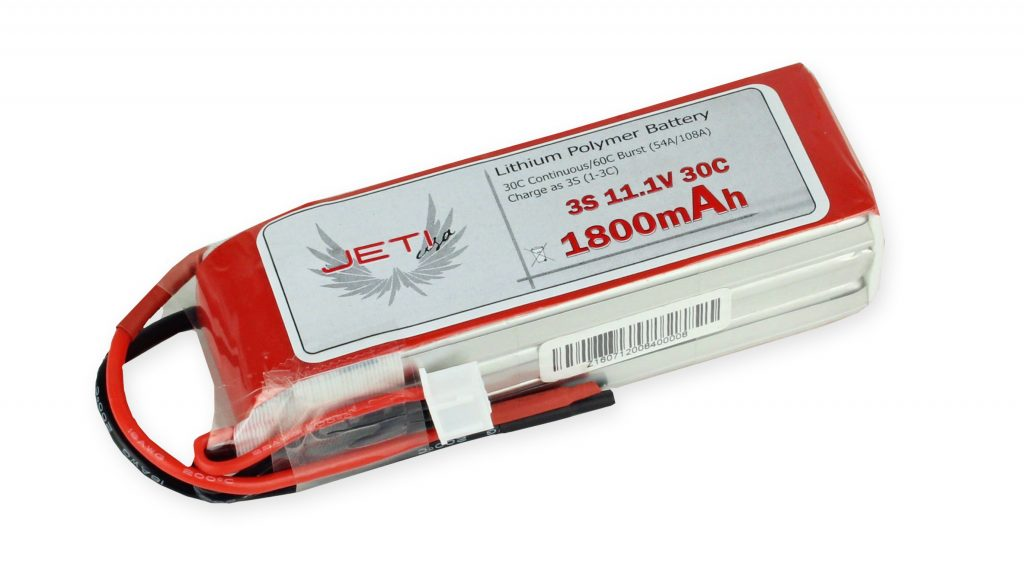## Tips From Our Techs: Battery C-Rating

With all the high-tech devices out there, we sometimes tend to over look the basics of the hobby. One common question we get is about the “C-Rating” on batteries and how to determine what you need.

C-rating is the number used to express the discharge and charge capability of a battery. Knowing how to calculate this becomes important when you are looking to outfit your model with the correct battery and space is a limiting factor.

To get the C-Rating appropriate for your aircraft use the following formula:

C-Rating needed = [Current Draw]/[Capacity (A)]

This will yield you the MINIMUM C-Rating that will work safely in your system. It is always a good idea to allow yourself a bit of overhead on your battery choice.

Lets break it down in the following examples.

Here is what you know: Your system will pull 90 amps, but you can only fit an 1800mAh 6S size battery pack. So your formula will look like this:

C-Rating needed = 90A/[1.8A]

C-Rating needed = 50C

*Take note that the capacity of your battery must be calculated in amps, not miliamps.

Here is another example where we are using a battery in series.

Here is what you know: You are flying a helicopter drawing 200 amps and using two 5000mAh 6S batteries.

C-Rating needed =200A/[5A]

C-Rating needed = 40C